# Yolo: v1-v3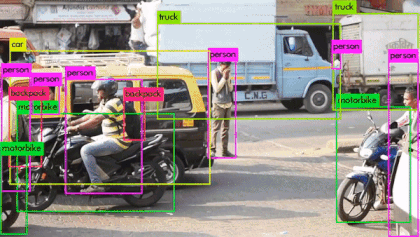# YOLO-v1YOLO-v1算法流程如上图所示：输入图片，resize到固定 448 * 448, 经过卷积网络得到网络输出，最后进行非极大值抑制得到最终结果. 整个流程相对RCNN系列更简洁.

## Unified Detection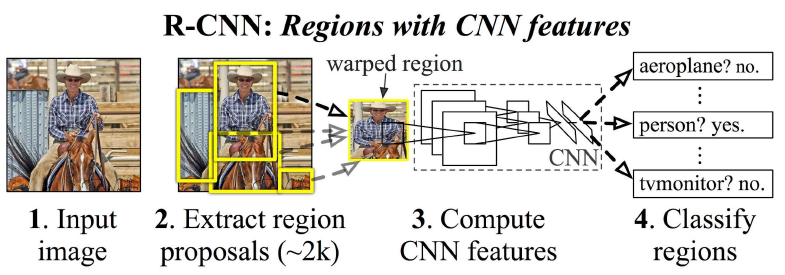YOLO 将目标检测的各个部分统一为一个网络. 使用整张图像的特征来预测所有的bounding box. 它同时预测类别与bounding box. 如上图所示.

## network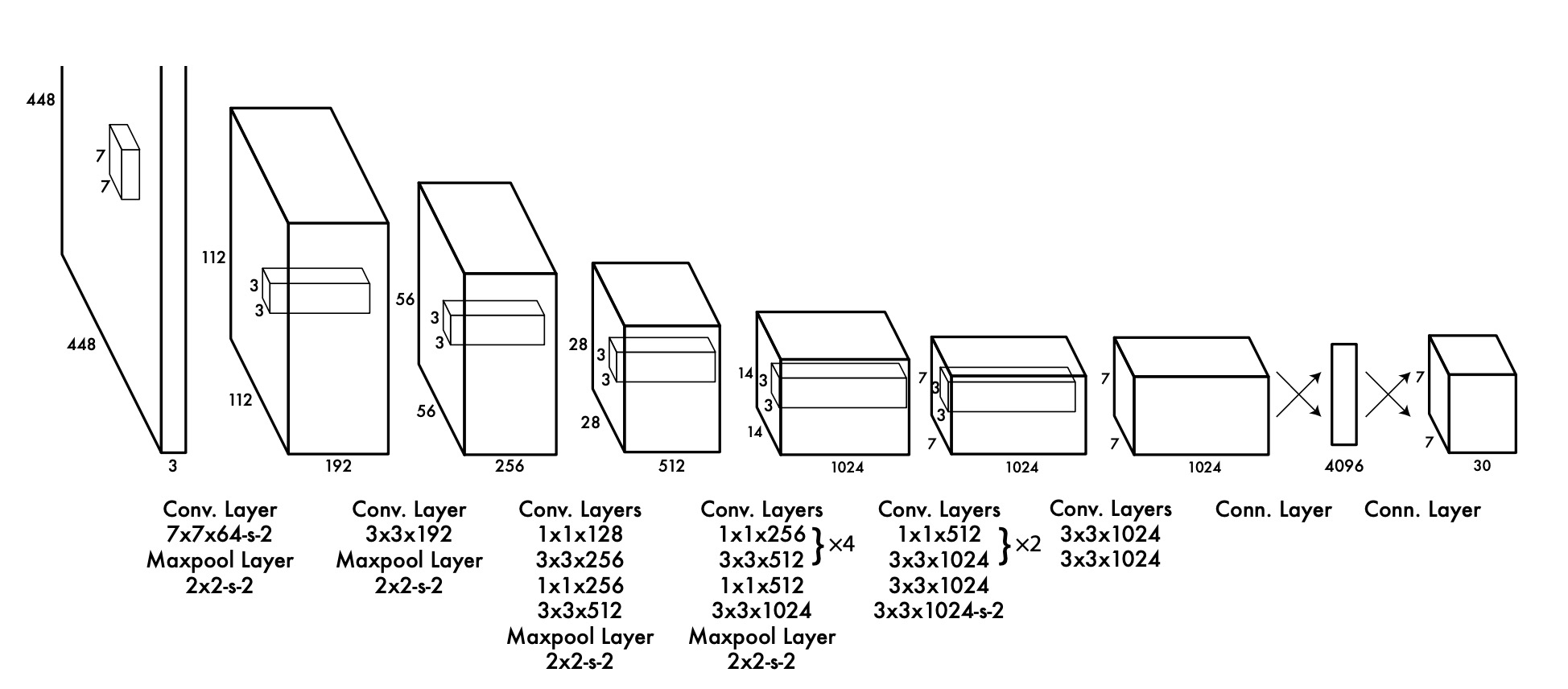yolo-v1 网络结构如上图所示. 它包含了24个卷积层和两个全连接层. 1 * 1 卷积用来降维. 在PASCAL VOC detection dataset背景下，网络最后输出为 7 * 7 * 30 的向量.

## 流程• $x, y$ 坐标表示预测bounding-box的中心点. 实际训练过程中, $x, y$ 是 bounding-box 中心位置相对于当前 grid 的偏移量.
• $w, h$ 是bounding-box 的宽度和高度. 实际训练过程中, 除以图像高度或宽度以归一化.
• $confidence$ 代表预测的bounding-box与 gt 之间的 IOU. $confidence$ 为 $Pr(Object) * IOU _{pred} ^{truth}$. 当框内有物体时, $Pr(Object)$ 为 1, 否则为 0.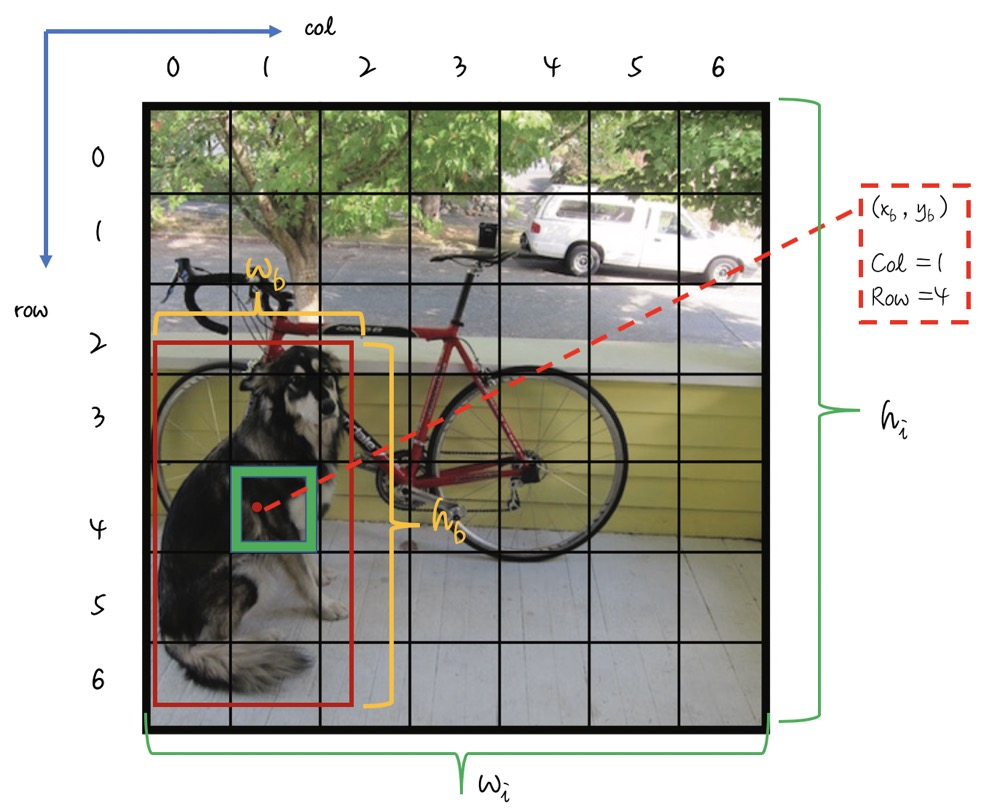$x, y, w, h$ 归一化说明如上图. 记 bounding-box 在原图的中心坐标位置为 $x_b, y_b$, 图像高度、宽度分别为 $h_i, w_i$, bounding-box 的高度和宽度分别为 $h_b, w_b$. 将原图分为 $S * S$ 个grid, 编号为 (0 … 6). 如当前预测狗的grid 为 (row=4, col=1). 转换公式如下：

$$x = \frac{x_b} { w_i} * S - col$$ $$y = \frac{y_b }{ h_i} * S - row$$ $$w = \frac{w_b}{w_i}$$ $$h = \frac{h_b}{h_i}$$

yolo-v1 输出如下图所示: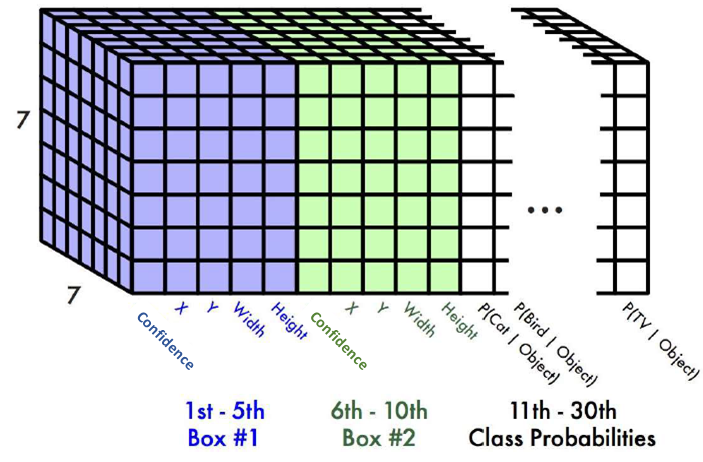## Loss Function• $(x,y)$ : 预测bounding-box 的 $x, y$坐标被参数化为特定网格单元位置的偏移，因此它们也被限定在0和1之间。只有当存在目标时，计算平方误差和. $\lambda _{coord}$ 提升bounding-box损失.
• $(w, h)$: bounding-box的宽和高, 归一化到 0-1 . 只有存在目标时才计算.
• $C_i$ : 在每个图像中，许多 grid 不包含任何对象. 这会将这些单元格的 $confidence$ 推向零，通常会超过包含对象的 grid 的梯度，从而使模型不稳定. 引入 $\lambda _{noobj}$ 系数控制比例.
• $p_i ( c)$ : 类别概率. 平方误差和.

## Other details

• Pascal voc下, 每张图片可以预测7 * 7 * 2 = 98 个bounding-box.
• yolo-v1 每个grid 只能预测两个box, 且属于同一类. 故对靠的近的不同类物体检测能力不足. 同时, 由于一个 grid cell 内的两个检测器都针对于同一个目标, 在训练时谁预测的结果与 GT 的IOU 最大谁就负责检测.
• 泛化能力较差. 倾向于检测长宽比类似于训练集长宽比的物体.

# YOLO-v2

yolo-v2 在yolo-v1 的基础上作了改进, 在保持处理速度的基础上， 分别从预测精度(Better)、检测速度(Faster)和识别更多目标(Stronger)上进行了优化. 本文只挑重点介绍.

### 3: Convolutional With Anchor Boxes

yolo-v2 移除了全连接层. 输入resize 到 416 * 416 , 输出特征图大小为 13 * 13. 每个 grid 预测 $N$(5) 个 anchor box. 且每个box 独享一套类别概率. 如下图:### 5: Direct location prediction

Faster R-CNN 中anchor-box 回归公式如下: $$t_x = (x -x_a)/ w_a, t_x = (y -y_a)/ h_a,$$ $$t_w = log(w/w_a), t_h = log(h / h_a),$$ $$t _x ^* = (x^* -x_a)/ w_a, t _y ^* = (y^* -y_a)/ h_a$$ $$t _w ^* = log(w^* / w_a), t _w ^* = log(w^* / w_a)$$

$x, y, w, h$ 分别为 box 的中心坐标与宽高. $x, x_a, x^*$ 分别为预测的box, anchor-box 以及 ground-truth box. loss 计算为预测值与anchor-box的偏移与ground-truth与anchor-box偏移的差值.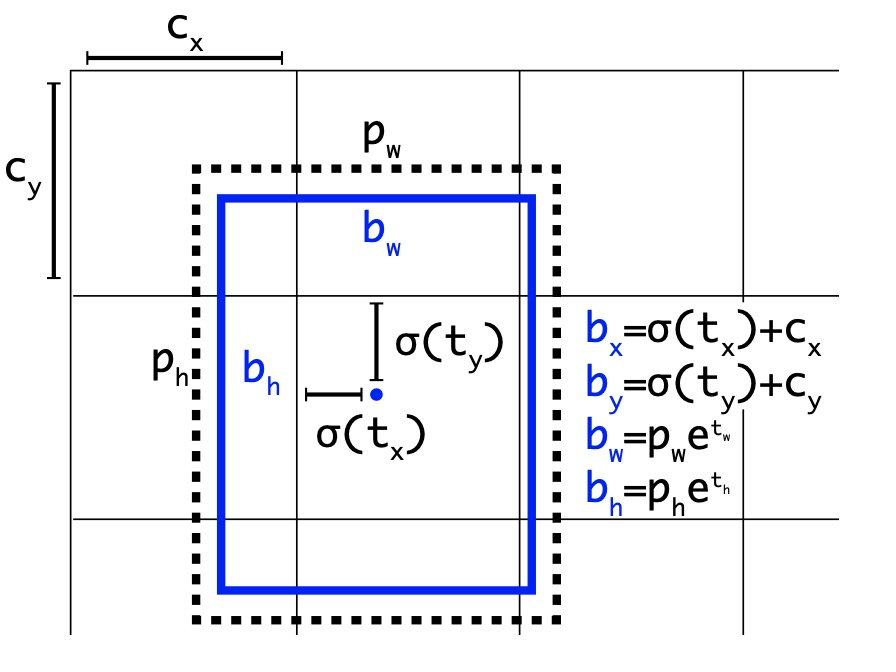yolo作者直接预测anchor-box 的位置, 而不再是与ground-truth之间的差值. 如上图所示, 网络最后输出的为 $13 * 13$ 的特征图, 图中的每一个 grid cell 仅仅是一个像素点而已. 对应到上图中每个 grid cell 的索引是其左上角像素的索引. 每个 grid cell 的尺度为1. 上图中grid cell 索引为$[0:12] * [0:12]$ .

$c_x, c_y$ 为当前grid cell的索引, $t_x, t_y$ 被约束到(0, 1)之间. 一是加快收敛, 其二保证中心约束在当前 grid cell内, 保证 box 中心落在哪个 grid cell 内,哪个负责检测.

YOLO-v2 每个 grid cell 设置了 5 个 anchor box, 在训练时 ground-truth 与哪一个 anchor box 的IOU 最大, 谁就负责检测该目标.

### 6: Darkent-19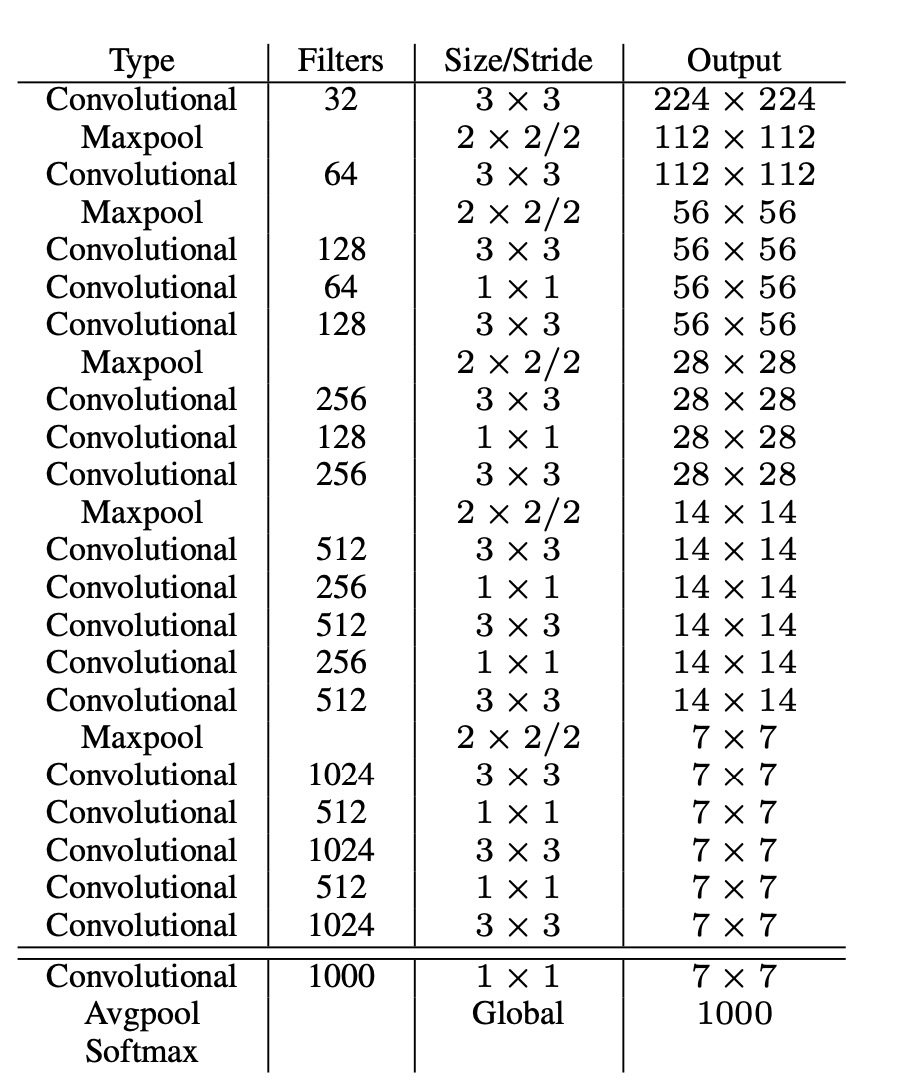# YOLO-v3

YOLO-v3 主要在 yolo-v2 上增加了多尺度, 提升了对小目标的检测能力. yolo-v3 主要从以下几方面做了改进.

## 1: Bounding box prediction

yolo-v3 对每个bounding-box 使用逻辑斯特回归预测是否包含物体.将与gt IOU 最大的先验框此项设为1. 如果先验框和 gt 的IOU 不是最佳的但是超过一定的阈值(文中设为0.5)则忽略此预测. 即不计入 loss. 对每一个 gt 只分配一个先验框, 如果一个先验框没有被分配任何 GT, 那么不计算此框不计算坐标预测和分类损失, 只计算 objectness 的损失.

## 3: Predictions Across Scales

yolo-v3 分别通过将输入图像的尺寸分别下采样32、16和8 倍, 输出各个尺度的检测结果. 每个尺度下分别分配三个长宽比的 anchor box, 其最小尺度分配最大的三个anchor box, 以此类推.

1: 第一次检测是由第82层进行的, 输出特征图大小为 13 x 13: 对应到原图, 如下图2: 第二次检测由第94层进行, 输出特征图大小为 26* 26. 负责检测中等大小的目标, 一共可输出 26 * 26 * 3 = 2028 个 box. 如下图: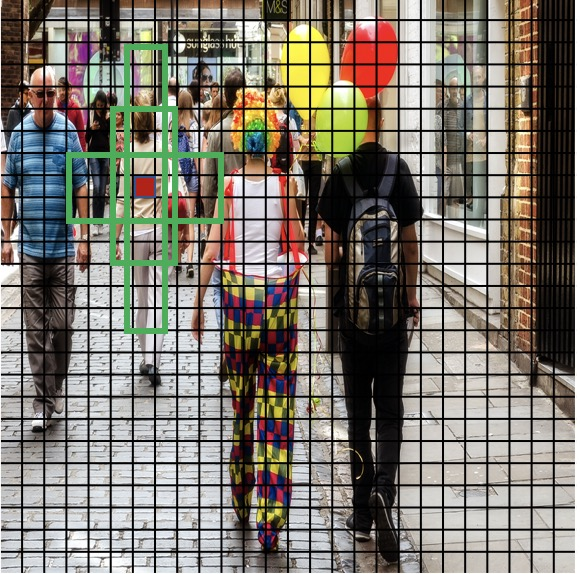3: 第三次检测由第 106 层进行, 输出特征图为 52 * 52. 主要负责检测小目标, 因此分配最小的anchor-box. 一共可输出 52 * 52 * 3 = 8112 个box.yolo-v3 在三个尺度下共输出bounding box 10647个, 预测的 box 数量超过 yolo v2的10X, 当然速度会慢一些.

## 4: Darknet 53

yolo-v2 使用的是darknet-19, v3中提出了 darknet-53 作为特征提取网络. 结构如下: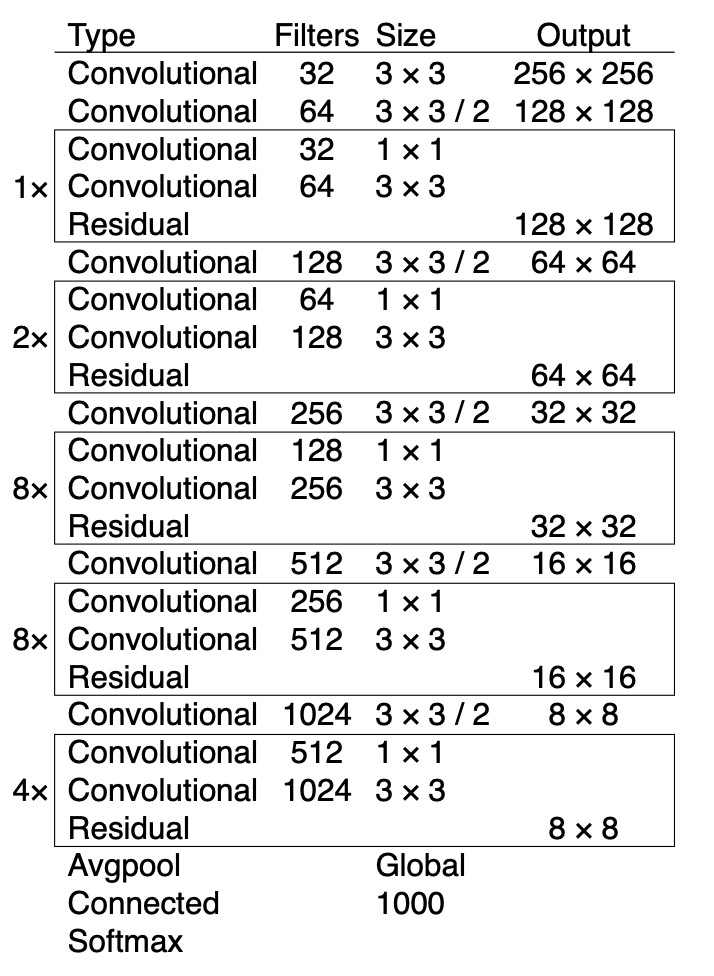## 5: yolo-v3 网络图